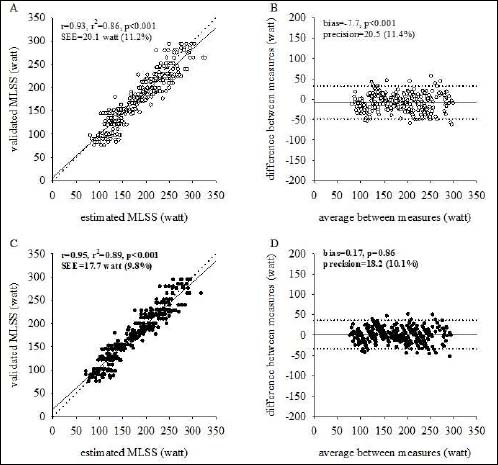Figure 1. Left panels: Individual validated Maximum Lactate steady-state (MLSS) values are plotted as a function of estimated values based on the original equation proposed by Fontana MLSS (○, panel A) and the new equation that incorporates age, sex and the trial absolute load (●, panel C). The identity (dashed) and the regression (solid) lines are displayed along with the coefficient of determination. Right panels: Individual differences between the values of validated MLSS and estimated values based on the original equation proposed by Fontana MLSS (○, panel B) and the new equation (●, panel D) are plotted as a function of the average of the two measures. The solid lines correspond to the average difference between measures (i.e. bias) while the dashed lines correspond to the limits of agreement.